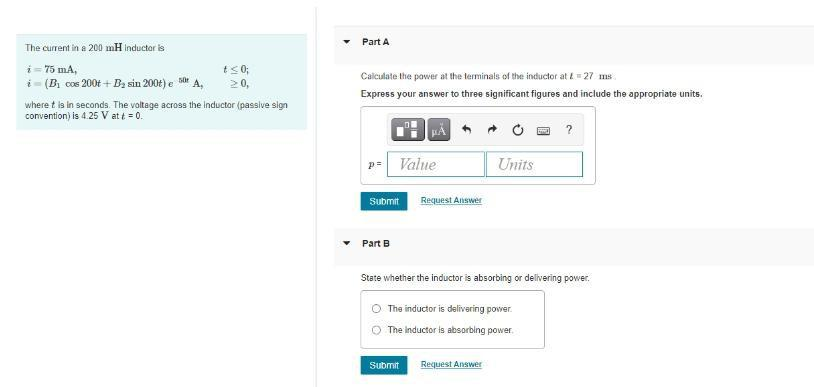Question:

# Part A The current in a 200 mH Inductaris i = 75 mA, t<0; i - (B, Cos 200++D, sin 200t) e 50e A, > 0, where t is in seconds. ThePart A The current in a 200 mH Inductaris i = 75 mA, t<0; i - (B, Cos 200++D, sin 200t) e 50e A, > 0, where t is in seconds. The voltage across the Inductor (passive sign convention) is 425 V at t = 0. Calculate the power at the terminals of the inductor at t = 27 ms Express your answer to three significant figures and include the appropriate units. ? Pa Value Units Submit Request Answer Part B State whether the inductor is absorbing or delivering power. The inductor is delivering power The inductor is absorbing power Submit Request Answer